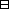## Group Theory and Linear Algebra

Last updated: 24 September 2014

## Lecture 27: Proof of the Orbit-Stabilizer theorem

Let $G$ be a group and let $S$ be a $G\text{-set.}$

 (a) The orbits partition $S\text{.}$ (b) If $s\in S$ and $H=\text{Stab}\left(s\right)$ then $φ: GH ⟶ Gs gH ⟼ gs$ is a function and $\phi$ is a bijection.

Let $G$ be a group and let $N$ be a subgroup of $G\text{.}$

 (a) The cosets in $G}{N}$ partition $G\text{.}$ (b) All cosets have the same size.Idea of proof. Let $g\in G\text{.}$ To show: $φ: gN ⟶ N gn ⟼ n$ is a function and $\phi$ is a bijection. $\square$

Let $\sim$ be an equivalence relation on a set $S\text{.}$

The equivalence classes partition $S\text{.}$Proof of the first Proposition.

(a)
To show: The orbits partition $S\text{.}$
To show:
 (aa) $\bigcup _{s\in S}Gs=S\text{.}$ (bb) If ${s}_{1},{s}_{2}\in S$ and $G{s}_{1}\cap G{s}_{2}\ne \varnothing$ then $G{s}_{1}=G{s}_{2}\text{.}$
(aa)
To show:
 (aaa) $\bigcup _{s\in S}Gs\subseteq S\text{.}$ (aab) $S\subseteq \bigcup _{s\in S}Gs\text{.}$
 (aaa) Since $Gs\subseteq S$ then $\bigcup _{s\in S}Gs\subseteq S\text{.}$ (aab) To show: If $a\in S$ then $a\in \bigcup _{s\in S}Gs\text{.}$ Since $a\in S,$ and $a\in Ga,$ then $a\in \bigcup _{s\in S}Gs\text{.}$ So $S\subseteq \bigcup _{s\in S}Gs\text{.}$
(ab) Assume ${s}_{1},{s}_{2}\in S$ and $G{s}_{1}\cap G{s}_{2}\ne \varnothing \text{.}$
To show: $G{s}_{1}=G{s}_{2}\text{.}$
Since $G{s}_{1}\cap G{s}_{2}\ne \varnothing$ there exists $t\in G{s}_{1}\cap G{s}_{2}\text{.}$
So there exist ${g}_{1},{g}_{2}\in G$ such that $g1s1=t=g2s2.$ So ${s}_{1}={g}_{1}^{-1}{g}_{2}{s}_{2}$ and ${s}_{2}={g}_{2}^{-1}{g}_{1}{s}_{1}\text{.}$
To show:
 (aba) $G{s}_{1}\subseteq G{s}_{2}\text{.}$ (abb) $G{s}_{2}\subseteq G{s}_{1}\text{.}$
 (aba) To show: If $\ell \in G{s}_{1}$ then $\ell \in G{s}_{2}\text{.}$ Assume $\ell \in G{s}_{1}\text{.}$ Then there exists $h\in G$ such that $\ell =h{s}_{1}\text{.}$ So $\ell =h{s}_{1}=h{g}_{1}^{-1}{g}_{2}{s}_{2}\in G{s}_{2},$ since $h{g}_{1}^{-1}{g}_{2}\in G\text{.}$ So $G{s}_{1}\subseteq G{s}_{2}\text{.}$ (abb) To show: If $m\in G{s}_{2}$ then $m\in G{s}_{1}\text{.}$ Assume $m\in G{s}_{2}\text{.}$ Then there exists $k\in G$ such that $m=k{s}_{2}\text{.}$ So $m=k{s}_{2}=k{g}_{2}^{-1}{g}_{1}{s}_{1}\in G{s}_{1},$ since $k{g}_{2}^{-1}{g}_{1}\in G\text{.}$ So $G{s}_{2}\subseteq G{s}_{1}\text{.}$ So $G{s}_{1}=G{s}_{2}\text{.}$ So the orbits partition $G\text{.}$
(b)
To show:
 (ba) $\begin{array}{cccc}\phi :& G}{H}& ⟶& Gs\\ & gH& ⟼& gs\end{array}$ is a function. (bb) $\phi$ is a bijection.
(ba) To show: If ${g}_{1}H,{g}_{2}H\in G}{H}$ and ${g}_{1}H={g}_{2}H$ then $\phi \left({g}_{1}H\right)=\phi \left({g}_{2}H\right)\text{.}$
Assume ${g}_{1},{g}_{2}\in G$ and ${g}_{1}H={g}_{2}H\text{.}$
Then ${g}_{1}\in {g}_{2}H\text{.}$
So there exists $h\in H$ with ${g}_{1}={g}_{2}h\text{.}$
To show: $\phi \left({g}_{1}H\right)=\phi \left({g}_{2}H\right)\text{.}$
To show: ${g}_{1}s={g}_{2}s\text{.}$ $g1s= g2hs=g2s,$ since $h\in \text{Stab}\left(s\right)\text{.}$
(bb)
To show: $\phi$ is a bijection.
To show: $\begin{array}{cccc}\psi :& Gs& ⟶& G}{H}\\ & t& ⟼& gH\end{array}$ where $g\in G$ such that $t=gs$ is an inverse function to $\phi \text{.}$
To show:
 (bba) If ${g}_{1},{g}_{2}\in G$ and ${g}_{1}s={g}_{2}s$ then $\psi \left({g}_{1}s\right)=\psi \left({g}_{2}s\right)\text{.}$ (bbb) $\phi \circ \psi ={\text{id}}_{G}{H}}$ and $\psi \circ \phi ={\text{id}}_{Gs}\text{.}$
(bba) Assume ${g}_{1},{g}_{2}\in G$ and ${g}_{1}s={g}_{2}s\text{.}$
Then ${g}_{1}^{-1}{g}_{2}s=s,$ so that ${g}_{1}^{-1}{g}_{2}\in \text{Stab}\left(s\right)\text{.}$
To show: $\psi \left({g}_{1}s\right)=\psi \left({g}_{2}s\right)\text{.}$
To show: ${g}_{1}H={g}_{2}H\text{.}$
To show:
 (bbaa) ${g}_{1}H\subseteq {g}_{2}H\text{.}$ (bbab) ${g}_{2}H\subseteq {g}_{1}H\text{.}$
 (bbaa) To show: If $x\in {g}_{1}H$ then $x\in {g}_{2}H\text{.}$ Assume $x\in {g}_{1}H\text{.}$ Then there exists $h\in H$ such that $x={g}_{1}h\text{.}$ To show: $x\in {g}_{2}h\text{.}$ $x=g1h=g2 g2-1g1h∈ g2H,$ since ${g}_{2}^{-1}{g}_{1}\in \text{Stab}\left(s\right)=H\text{.}$ So ${g}_{1}H\subseteq {g}_{2}H\text{.}$ (bbab) To show: If $y\in {g}_{2}H$ then $y\in {g}_{1}H\text{.}$ Assume $y\in {g}_{2}H\text{.}$ Then there exists $k\in H$ such that $y={g}_{2}k\text{.}$ So $y=g2k=g1g1-1g2k ∈g1H,$ since ${g}_{1}^{-1}{g}_{2}\in \text{Stab}\left(s\right)=H\text{.}$ So ${g}_{2}H\subseteq {g}_{1}H\text{.}$
So ${g}_{2}H={g}_{1}H\text{.}$
(bbb) To show: $\phi \circ \psi ={\text{id}}_{G}{H}}$ and $\psi \circ \phi ={\text{id}}_{Gs}\text{.}$
If $g\in G$ then $(φ∘ψ)(gH)= φ(ψ(gH))= φ(gs)=gH$ and $(ψ∘φ)(gs)= ψ(φ(gs))= ψ(gH)=gs.$ So $\phi \circ \psi ={\text{id}}_{G}{H}}$ and $\psi \circ \phi ={\text{id}}_{Gs}\text{.}$

$\square$

## Notes and References

These are a typed copy of Lecture 27 from a series of handwritten lecture notes for the class Group Theory and Linear Algebra given on October 7, 2011.